## Fermat's Little Theorem

If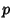is a Prime number anda Natural Number, then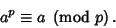(1)

Furthermore, if(does not divide), then there exists some smallest exponentsuch that(2)

anddivides. Hence,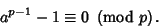(3)

This is a generalization of the Chinese Hypothesis and a special case of Euler's Theorem. It is sometimes called Fermat's Primality Test and is a Necessary but not Sufficient test for primality. Although it was presumably proved (but suppressed) by Fermat,the first proof was published by Eulerin 1749.

The theorem is easily proved using mathematical Induction. Suppose. Then examine(4)

From the Binomial Theorem,(5)

Rewriting,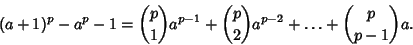(6)

Butdivides the right side, so it also divides the left side. Combining with the induction hypothesis gives thatdivides the sum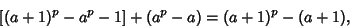(7)

as assumed, so the hypothesis is true for any. The theorem is sometimes called Fermat's Simple Theorem. Wilson's Theorem follows as a Corollary of Fermat's Little Theorem.

Fermat's little theorem shows that, ifis Prime, there does not exist a base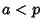with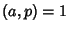such thatpossesses a nonzero residue modulo. If such baseexists,is therefore guaranteed to be composite. However, the lack of a nonzero residue in Fermat's little theorem does not guarantee thatis Prime. The property of unambiguously certifying composite numbers while passing some Primes make Fermat's little theorem a Compositeness Test which is sometimes called the Fermat Compositeness Test. Composite Numbers known as Fermat Pseudoprimes (or sometimes simply Pseudoprimes'') have zero residue for somes and so are not identified as composite. Worse still, there exist numbers known as Carmichael Numbers (the smallest of which is 561) which give zero residue for any choice of the baseRelatively Prime to. However, Fermat's Little Theorem Converse provides a criterion for certifying the primality of a number.

A number satisfying Fermat's little theorem for some nontrivial base and which is not known to be composite is called a Probable Prime. A table of the smallest Pseudoprimes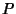for the first 100 basesfollows (Sloane's A007535).2 341 22 69 42 205 62 63 82 91 3 91 23 33 43 77 63 341 83 105 4 15 24 25 44 45 64 65 84 85 5 124 25 28 45 76 65 133 85 129 6 35 26 27 46 133 66 91 86 87 7 25 27 65 47 65 67 85 87 91 8 9 28 87 48 49 68 69 88 91 9 28 29 35 49 66 69 85 89 99 10 33 30 49 50 51 70 169 90 91 11 15 31 49 51 65 71 105 91 115 12 65 32 33 52 85 72 85 92 93 13 21 33 85 53 65 73 111 93 301 14 15 34 35 54 55 74 75 94 95 15 341 35 51 55 63 75 91 95 141 16 51 36 91 56 57 76 77 96 133 17 45 37 45 57 65 77 95 97 105 18 25 38 39 58 95 78 341 98 99 19 45 39 95 59 87 79 91 99 145 20 21 40 91 60 341 80 81 100 259 21 55 41 105 61 91 81 85

See also Binomial Theorem, Carmichael Number, Chinese Hypothesis, Composite Number, Compositeness Test, Euler's Theorem, Fermat's Little Theorem Converse, Fermat Pseudoprime, Modulo Multiplication Group, Pratt Certificate, Primality Test, Prime Number, Pseudoprime, Relatively Prime, Totient Function, Wieferich Prime, Wilson's Theorem, Witness

References

Ball, W. W. R. and Coxeter, H. S. M. Mathematical Recreations and Essays, 13th ed. New York: Dover, p. 61, 1987.

Conway, J. H. and Guy, R. K. The Book of Numbers. New York: Springer-Verlag, pp. 141-142, 1996.

Courant, R. and Robbins, H. Fermat's Theorem.'' §2.2 in Supplement to Ch. 1 in What is Mathematics?: An Elementary Approach to Ideas and Methods, 2nd ed. Oxford, England: Oxford University Press, pp. 37-38, 1996.

Shanks, D. Solved and Unsolved Problems in Number Theory, 4th ed. New York: Chelsea, p. 20, 1993.

Sloane, N. J. A. Sequence A007535/M5440 in An On-Line Version of the Encyclopedia of Integer Sequences.'' http://www.research.att.com/~njas/sequences/eisonline.html and Sloane, N. J. A. and Plouffe, S. The Encyclopedia of Integer Sequences. San Diego: Academic Press, 1995.